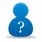Welcome Guest.
You haven't logged in yet. If you don't have an account you can register now.

## bigal_nz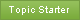635 posts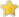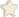Ultimate Geek
Inactive user

#32879 26-Apr-2009 12:21

Hi Guys,

Just trying to help out a family member who is with Orcon and been having no end of trouble.

When I started to look at his connection I immediately noticed a few things:

(a) His house wiring was terrible (excess lengths of cable under the house etc (using that flat crapy cable)
(b) lots of Timeouts on a C:\ping www.google.co.nz -n 1000 (only about 90% of packets came back)
(c) Fairly low sync speed considering ADSL2+ and distance from exchange (about 3.5-4km)

So in order to isolate the fault the wires were cut at the Demarc and wired the modem in directly to the street. I really thought this would make a huge difference, but alas no - there was still heaps of ping drop outs, and the sync speed didnt go up - > this implies the problem lies between the Demarc and the exchange?

Orcons initial response was to up the SNR Margin to 12 - which I hate ISP's doing - thats just masking the poor connection by slowing us down!

So after many phone calls to Orcon they finally agree to send out a Chorus tech. Now I wasnt around when he came but I rang him afterwards. He said he spent about an hour at the house and had no disconnections and so he left. He said he was paid by the hour and could not afford to spend any more time on it. He went on to say some of the Chorus techs are not paid by the hour and to try to get one of them onto it!!!

Ping statistics for 66.102.7.99:
Packets: Sent = 1000, Received = 975, Lost = 25 (2% loss),
Approximate round trip times in milli-seconds:
Minimum = 162ms, Maximum = 2102ms, Average = 175ms

Here is some screen shots from DMT tool - but I really dont know how to interpret them - perhaps someone else can help here:Cheers

-Al
PS: Anyone know who the best Chorus ADSL techs are in Auckland?

## Norton Secure VPN

Affiliate link: Norton Secure VPN helps secure private information using bank-grade encryption when using public Wi-Fi on your PC, Mac, or mobile device.

## Ilmarin

94 postsMaster Geek

Looks fine to me. 5364 kbps is pretty decent for 48 dB attenuation... I used to get that on 42 dB attenuation. Don't know why Orcon would change the SNR margin if there were no complaints of poor connection stability, but then 12 dB is standard. DMT Tool might be able to lower it if your family member wants more speed and the modem supports it, although going below 8 dB does tend to push the stability a bit.

2% packetloss to the US is nothing unusual for Orcon, even if they are only ICMP ping packets of default size. Packetloss is particularly bad during peak hours due to congestion on Orcon's oversubscribed international links. In any case, it has nothing to do with anything between the modem and the exchange.

What exactly has your family member been complaining about? If it's poor international performance during peak hours, then he's basically in the same boat as every other Orcon customer.

## Tzleren

30 postsGeek

5.2Mb/1Mb @ 48dB is definitely acceptable.

## bigal_nz635 postsUltimate Geek
Inactive user

Well another unusual thing I have noticed is the SNR margin seems to flucuate the longer the modem is on, and generally in a downward trend. Sometime it hits zero then goes to a crazy figure like 236212.

Sometimes it can take half a minute to load a webpage etc etc etc.

-Al

## cyril7

8731 postsUber Geek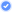ID Verified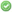TrustedSubscriber

Can I recommend that you force the modem back to ADSL1, with a line length over 3.5km there is no speed advantage in ADSL2+ and by reverting to ADSL1 you circumvent any issues that ADLS2 does with regard to dynamic power control that could be why you get a spiralling S/N issue.

Beyond that I think at 48dB and 5Mb/s you are doing pretty good.

Cyril

## orconuser121

43 postsGeek

Can this application be used on any ADSL router? I have a Dynalink RTA 1320, yet when I try to connect, It doesn't work!

## bigal_nz635 postsUltimate Geek
Inactive user

2% packetloss to the US is nothing unusual for Orcon, even if they are only ICMP ping packets of default size. Packetloss is particularly bad during peak hours due to congestion on Orcon's oversubscribed international links. In any case, it has nothing to do with anything between the modem and the exchange.

Yeah but I still get lost packts on the way to the orcon dns server!!! I can accept it might be reasonable to loose a few packets on the way to google - but Orcons own DNS server.....

-Al

## Ilmarin

94 postsMaster Geek

Do you get packetloss to the DNS server during peak hours, or both peak and off-peak? Either way it would be somewhat unusual... and worth sending a traceroute to Orcon so that they can check the relevant routers.

## orconuser121

43 postsGeek

Microsoft Windows XP [Version 5.1.2600]

Pinging www.l.google.com [66.102.7.99] with 32 bytes of data:

Reply from 66.102.7.99: bytes=32 time=150ms TTL=243
Reply from 66.102.7.99: bytes=32 time=148ms TTL=243
Reply from 66.102.7.99: bytes=32 time=152ms TTL=243
Reply from 66.102.7.99: bytes=32 time=149ms TTL=243

Ping statistics for 66.102.7.99:
Packets: Sent = 4, Received = 4, Lost = 0 (0% loss),
Approximate round trip times in milli-seconds:
Minimum = 148ms, Maximum = 152ms, Average = 149ms

This is the ORCON DNS PING:
C:\Documents and Settings\Terry>ping 60.234.1.1

Pinging 60.234.1.1 with 32 bytes of data:

Reply from 60.234.1.1: bytes=32 time=25ms TTL=60
Reply from 60.234.1.1: bytes=32 time=24ms TTL=60
Reply from 60.234.1.1: bytes=32 time=24ms TTL=60
Reply from 60.234.1.1: bytes=32 time=24ms TTL=60

Ping statistics for 60.234.1.1:
Packets: Sent = 4, Received = 4, Lost = 0 (0% loss),
Approximate round trip times in milli-seconds:
Minimum = 24ms, Maximum = 25ms, Average = 24ms

No problems there....

## bigal_nz635 postsUltimate Geek
Inactive user

orconuser121: Microsoft Windows XP [Version 5.1.2600]

Pinging www.l.google.com [66.102.7.99] with 32 bytes of data:

Reply from 66.102.7.99: bytes=32 time=150ms TTL=243

Reply from 66.102.7.99: bytes=32 time=148ms TTL=243

Reply from 66.102.7.99: bytes=32 time=152ms TTL=243

Reply from 66.102.7.99: bytes=32 time=149ms TTL=243

Ping statistics for 66.102.7.99:

Packets: Sent = 4, Received = 4, Lost = 0 (0% loss),

Approximate round trip times in milli-seconds:

Minimum = 148ms, Maximum = 152ms, Average = 149ms

This is the ORCON DNS PING:

C:\Documents and Settings\Terry>ping 60.234.1.1

Pinging 60.234.1.1 with 32 bytes of data:

Reply from 60.234.1.1: bytes=32 time=25ms TTL=60

Reply from 60.234.1.1: bytes=32 time=24ms TTL=60

Reply from 60.234.1.1: bytes=32 time=24ms TTL=60

Reply from 60.234.1.1: bytes=32 time=24ms TTL=60

Ping statistics for 60.234.1.1:

Packets: Sent = 4, Received = 4, Lost = 0 (0% loss),

Approximate round trip times in milli-seconds:

Minimum = 24ms, Maximum = 25ms, Average = 24ms

No problems there....

Try ping again with "-n 100" tacked on the end

## orconuser121

43 postsGeek

C:\Documents and Settings\Terry>ping 60.234.1.1 -n 100

Pinging 60.234.1.1 with 32 bytes of data:

Reply from 60.234.1.1: bytes=32 time=22ms TTL=60
Reply from 60.234.1.1: bytes=32 time=26ms TTL=60
Reply from 60.234.1.1: bytes=32 time=25ms TTL=60
Reply from 60.234.1.1: bytes=32 time=23ms TTL=60
Reply from 60.234.1.1: bytes=32 time=21ms TTL=60
Reply from 60.234.1.1: bytes=32 time=24ms TTL=60
Reply from 60.234.1.1: bytes=32 time=24ms TTL=60
Reply from 60.234.1.1: bytes=32 time=24ms TTL=60
Reply from 60.234.1.1: bytes=32 time=197ms TTL=60
Reply from 60.234.1.1: bytes=32 time=22ms TTL=60
Reply from 60.234.1.1: bytes=32 time=22ms TTL=60
Reply from 60.234.1.1: bytes=32 time=21ms TTL=60
Reply from 60.234.1.1: bytes=32 time=23ms TTL=60
Reply from 60.234.1.1: bytes=32 time=22ms TTL=60
Reply from 60.234.1.1: bytes=32 time=21ms TTL=60
Reply from 60.234.1.1: bytes=32 time=24ms TTL=60
Reply from 60.234.1.1: bytes=32 time=26ms TTL=60
Reply from 60.234.1.1: bytes=32 time=22ms TTL=60
Reply from 60.234.1.1: bytes=32 time=23ms TTL=60
Reply from 60.234.1.1: bytes=32 time=26ms TTL=60
Reply from 60.234.1.1: bytes=32 time=25ms TTL=60
Reply from 60.234.1.1: bytes=32 time=25ms TTL=60
Reply from 60.234.1.1: bytes=32 time=22ms TTL=60
Reply from 60.234.1.1: bytes=32 time=21ms TTL=60
Reply from 60.234.1.1: bytes=32 time=25ms TTL=60
Reply from 60.234.1.1: bytes=32 time=22ms TTL=60
Reply from 60.234.1.1: bytes=32 time=23ms TTL=60
Reply from 60.234.1.1: bytes=32 time=25ms TTL=60
Reply from 60.234.1.1: bytes=32 time=24ms TTL=60
Reply from 60.234.1.1: bytes=32 time=191ms TTL=60
Reply from 60.234.1.1: bytes=32 time=23ms TTL=60
Reply from 60.234.1.1: bytes=32 time=25ms TTL=60
Reply from 60.234.1.1: bytes=32 time=22ms TTL=60
Reply from 60.234.1.1: bytes=32 time=23ms TTL=60
Reply from 60.234.1.1: bytes=32 time=21ms TTL=60
Reply from 60.234.1.1: bytes=32 time=25ms TTL=60
Reply from 60.234.1.1: bytes=32 time=22ms TTL=60
Reply from 60.234.1.1: bytes=32 time=22ms TTL=60
Reply from 60.234.1.1: bytes=32 time=22ms TTL=60
Reply from 60.234.1.1: bytes=32 time=22ms TTL=60
Reply from 60.234.1.1: bytes=32 time=22ms TTL=60
Reply from 60.234.1.1: bytes=32 time=22ms TTL=60
Reply from 60.234.1.1: bytes=32 time=22ms TTL=60
Reply from 60.234.1.1: bytes=32 time=22ms TTL=60
Reply from 60.234.1.1: bytes=32 time=23ms TTL=60
Reply from 60.234.1.1: bytes=32 time=25ms TTL=60
Reply from 60.234.1.1: bytes=32 time=24ms TTL=60
Reply from 60.234.1.1: bytes=32 time=23ms TTL=60
Reply from 60.234.1.1: bytes=32 time=21ms TTL=60
Reply from 60.234.1.1: bytes=32 time=25ms TTL=60
Reply from 60.234.1.1: bytes=32 time=77ms TTL=60
Reply from 60.234.1.1: bytes=32 time=22ms TTL=60
Reply from 60.234.1.1: bytes=32 time=21ms TTL=60
Reply from 60.234.1.1: bytes=32 time=24ms TTL=60
Reply from 60.234.1.1: bytes=32 time=23ms TTL=60
Reply from 60.234.1.1: bytes=32 time=22ms TTL=60
Reply from 60.234.1.1: bytes=32 time=26ms TTL=60
Reply from 60.234.1.1: bytes=32 time=23ms TTL=60
Reply from 60.234.1.1: bytes=32 time=22ms TTL=60
Reply from 60.234.1.1: bytes=32 time=21ms TTL=60
Reply from 60.234.1.1: bytes=32 time=24ms TTL=60
Reply from 60.234.1.1: bytes=32 time=33ms TTL=60
Reply from 60.234.1.1: bytes=32 time=21ms TTL=60
Reply from 60.234.1.1: bytes=32 time=24ms TTL=60
Reply from 60.234.1.1: bytes=32 time=25ms TTL=60
Reply from 60.234.1.1: bytes=32 time=22ms TTL=60
Reply from 60.234.1.1: bytes=32 time=22ms TTL=60
Reply from 60.234.1.1: bytes=32 time=22ms TTL=60
Reply from 60.234.1.1: bytes=32 time=22ms TTL=60
Reply from 60.234.1.1: bytes=32 time=23ms TTL=60
Reply from 60.234.1.1: bytes=32 time=25ms TTL=60
Reply from 60.234.1.1: bytes=32 time=24ms TTL=60
Reply from 60.234.1.1: bytes=32 time=24ms TTL=60
Reply from 60.234.1.1: bytes=32 time=23ms TTL=60
Reply from 60.234.1.1: bytes=32 time=21ms TTL=60
Reply from 60.234.1.1: bytes=32 time=25ms TTL=60
Reply from 60.234.1.1: bytes=32 time=26ms TTL=60
Reply from 60.234.1.1: bytes=32 time=24ms TTL=60
Reply from 60.234.1.1: bytes=32 time=24ms TTL=60
Reply from 60.234.1.1: bytes=32 time=23ms TTL=60
Reply from 60.234.1.1: bytes=32 time=25ms TTL=60
Reply from 60.234.1.1: bytes=32 time=24ms TTL=60
Reply from 60.234.1.1: bytes=32 time=25ms TTL=60
Reply from 60.234.1.1: bytes=32 time=24ms TTL=60
Reply from 60.234.1.1: bytes=32 time=24ms TTL=60
Reply from 60.234.1.1: bytes=32 time=22ms TTL=60
Reply from 60.234.1.1: bytes=32 time=21ms TTL=60
Reply from 60.234.1.1: bytes=32 time=24ms TTL=60
Reply from 60.234.1.1: bytes=32 time=23ms TTL=60
Reply from 60.234.1.1: bytes=32 time=21ms TTL=60
Reply from 60.234.1.1: bytes=32 time=25ms TTL=60
Reply from 60.234.1.1: bytes=32 time=22ms TTL=60
Reply from 60.234.1.1: bytes=32 time=22ms TTL=60
Reply from 60.234.1.1: bytes=32 time=21ms TTL=60
Reply from 60.234.1.1: bytes=32 time=28ms TTL=60
Reply from 60.234.1.1: bytes=32 time=23ms TTL=60
Reply from 60.234.1.1: bytes=32 time=25ms TTL=60
Reply from 60.234.1.1: bytes=32 time=24ms TTL=60
Reply from 60.234.1.1: bytes=32 time=23ms TTL=60
Reply from 60.234.1.1: bytes=32 time=21ms TTL=60

Ping statistics for 60.234.1.1:
Packets: Sent = 100, Received = 100, Lost = 0 (0% loss),
Approximate round trip times in milli-seconds:
Minimum = 21ms, Maximum = 197ms, Average = 27ms

## orconuser121

43 postsGeek

C:\Documents and Settings\Terry>ping www.google.co.nz -n 100

Pinging www.l.google.com [66.102.7.99] with 32 bytes of data:

Reply from 66.102.7.99: bytes=32 time=151ms TTL=243
Reply from 66.102.7.99: bytes=32 time=151ms TTL=243
Reply from 66.102.7.99: bytes=32 time=152ms TTL=243
Reply from 66.102.7.99: bytes=32 time=148ms TTL=243
Reply from 66.102.7.99: bytes=32 time=151ms TTL=243
Reply from 66.102.7.99: bytes=32 time=151ms TTL=243
Reply from 66.102.7.99: bytes=32 time=149ms TTL=243
Reply from 66.102.7.99: bytes=32 time=147ms TTL=243
Reply from 66.102.7.99: bytes=32 time=148ms TTL=243
Reply from 66.102.7.99: bytes=32 time=151ms TTL=243
Reply from 66.102.7.99: bytes=32 time=151ms TTL=243
Reply from 66.102.7.99: bytes=32 time=151ms TTL=243
Reply from 66.102.7.99: bytes=32 time=150ms TTL=243
Reply from 66.102.7.99: bytes=32 time=150ms TTL=243
Reply from 66.102.7.99: bytes=32 time=152ms TTL=243
Reply from 66.102.7.99: bytes=32 time=149ms TTL=243
Reply from 66.102.7.99: bytes=32 time=147ms TTL=243
Reply from 66.102.7.99: bytes=32 time=147ms TTL=243
Reply from 66.102.7.99: bytes=32 time=149ms TTL=243
Reply from 66.102.7.99: bytes=32 time=148ms TTL=243
Reply from 66.102.7.99: bytes=32 time=151ms TTL=243
Reply from 66.102.7.99: bytes=32 time=151ms TTL=243
Reply from 66.102.7.99: bytes=32 time=148ms TTL=243
Reply from 66.102.7.99: bytes=32 time=153ms TTL=243
Reply from 66.102.7.99: bytes=32 time=152ms TTL=243
Reply from 66.102.7.99: bytes=32 time=151ms TTL=243
Reply from 66.102.7.99: bytes=32 time=149ms TTL=243
Reply from 66.102.7.99: bytes=32 time=150ms TTL=243
Reply from 66.102.7.99: bytes=32 time=148ms TTL=243
Reply from 66.102.7.99: bytes=32 time=150ms TTL=243
Reply from 66.102.7.99: bytes=32 time=149ms TTL=243
Reply from 66.102.7.99: bytes=32 time=149ms TTL=243
Reply from 66.102.7.99: bytes=32 time=149ms TTL=243
Reply from 66.102.7.99: bytes=32 time=149ms TTL=243
Reply from 66.102.7.99: bytes=32 time=149ms TTL=243
Reply from 66.102.7.99: bytes=32 time=148ms TTL=243
Reply from 66.102.7.99: bytes=32 time=150ms TTL=243
Reply from 66.102.7.99: bytes=32 time=148ms TTL=243
Reply from 66.102.7.99: bytes=32 time=152ms TTL=243
Reply from 66.102.7.99: bytes=32 time=150ms TTL=243
Reply from 66.102.7.99: bytes=32 time=148ms TTL=243
Reply from 66.102.7.99: bytes=32 time=152ms TTL=243
Reply from 66.102.7.99: bytes=32 time=149ms TTL=243
Reply from 66.102.7.99: bytes=32 time=149ms TTL=243
Reply from 66.102.7.99: bytes=32 time=149ms TTL=243
Reply from 66.102.7.99: bytes=32 time=149ms TTL=243
Reply from 66.102.7.99: bytes=32 time=149ms TTL=243
Reply from 66.102.7.99: bytes=32 time=148ms TTL=243
Reply from 66.102.7.99: bytes=32 time=149ms TTL=243
Reply from 66.102.7.99: bytes=32 time=151ms TTL=243
Reply from 66.102.7.99: bytes=32 time=150ms TTL=243
Reply from 66.102.7.99: bytes=32 time=149ms TTL=243
Reply from 66.102.7.99: bytes=32 time=147ms TTL=243
Reply from 66.102.7.99: bytes=32 time=149ms TTL=243
Reply from 66.102.7.99: bytes=32 time=148ms TTL=243
Reply from 66.102.7.99: bytes=32 time=152ms TTL=243
Reply from 66.102.7.99: bytes=32 time=152ms TTL=243
Reply from 66.102.7.99: bytes=32 time=151ms TTL=243
Reply from 66.102.7.99: bytes=32 time=149ms TTL=243
Reply from 66.102.7.99: bytes=32 time=148ms TTL=243
Reply from 66.102.7.99: bytes=32 time=151ms TTL=243
Reply from 66.102.7.99: bytes=32 time=150ms TTL=243
Reply from 66.102.7.99: bytes=32 time=148ms TTL=243
Reply from 66.102.7.99: bytes=32 time=149ms TTL=243
Reply from 66.102.7.99: bytes=32 time=149ms TTL=243
Reply from 66.102.7.99: bytes=32 time=151ms TTL=243
Reply from 66.102.7.99: bytes=32 time=153ms TTL=243
Reply from 66.102.7.99: bytes=32 time=148ms TTL=243
Reply from 66.102.7.99: bytes=32 time=149ms TTL=243
Reply from 66.102.7.99: bytes=32 time=150ms TTL=243
Reply from 66.102.7.99: bytes=32 time=149ms TTL=243
Reply from 66.102.7.99: bytes=32 time=149ms TTL=243
Reply from 66.102.7.99: bytes=32 time=150ms TTL=243
Reply from 66.102.7.99: bytes=32 time=150ms TTL=243
Reply from 66.102.7.99: bytes=32 time=152ms TTL=243
Reply from 66.102.7.99: bytes=32 time=148ms TTL=243
Reply from 66.102.7.99: bytes=32 time=149ms TTL=243
Reply from 66.102.7.99: bytes=32 time=148ms TTL=243
Reply from 66.102.7.99: bytes=32 time=151ms TTL=243
Reply from 66.102.7.99: bytes=32 time=148ms TTL=243
Reply from 66.102.7.99: bytes=32 time=150ms TTL=243
Reply from 66.102.7.99: bytes=32 time=151ms TTL=243
Reply from 66.102.7.99: bytes=32 time=151ms TTL=243
Reply from 66.102.7.99: bytes=32 time=151ms TTL=243
Reply from 66.102.7.99: bytes=32 time=152ms TTL=243
Reply from 66.102.7.99: bytes=32 time=151ms TTL=243
Reply from 66.102.7.99: bytes=32 time=150ms TTL=243
Reply from 66.102.7.99: bytes=32 time=148ms TTL=243
Reply from 66.102.7.99: bytes=32 time=154ms TTL=243
Reply from 66.102.7.99: bytes=32 time=149ms TTL=243
Reply from 66.102.7.99: bytes=32 time=151ms TTL=243
Reply from 66.102.7.99: bytes=32 time=151ms TTL=243
Reply from 66.102.7.99: bytes=32 time=150ms TTL=243
Reply from 66.102.7.99: bytes=32 time=148ms TTL=243
Reply from 66.102.7.99: bytes=32 time=152ms TTL=243
Reply from 66.102.7.99: bytes=32 time=150ms TTL=243
Reply from 66.102.7.99: bytes=32 time=153ms TTL=243
Reply from 66.102.7.99: bytes=32 time=152ms TTL=243
Reply from 66.102.7.99: bytes=32 time=149ms TTL=243
Reply from 66.102.7.99: bytes=32 time=149ms TTL=243

Ping statistics for 66.102.7.99:
Packets: Sent = 100, Received = 100, Lost = 0 (0% loss),
Approximate round trip times in milli-seconds:
Minimum = 147ms, Maximum = 154ms, Average = 149ms

## bigal_nz635 postsUltimate Geek
Inactive user

Well there is my point - why can he get no timeouts yet I get all these lost packets?

## orconuser121

43 postsGeek

Ping statistics for 212.58.254.252:
Packets: Sent = 1000, Received = 1000, Lost = 0 (0% loss),
Approximate round trip times in milli-seconds:
Minimum = 300ms, Maximum = 386ms, Average = 305ms

That one was for bbc.co.uk using -n 1000

News and reviews »

D-Link G415 4G Smart Router Review
Posted 27-Jun-2022 17:24

New Zealand Video Game Sales Reaches \$540 Million
Posted 26-Jun-2022 14:49

Github Copilot Generally Available to All Developers
Posted 26-Jun-2022 14:37

Logitech G Introduces the New Astro A10 Headset
Posted 26-Jun-2022 14:20

Fitbit introduces Sleep Profiles
Posted 26-Jun-2022 14:11

Synology Introduces FlashStation FS3410
Posted 26-Jun-2022 14:04

Intel Arc A380 Graphics First Available in China
Posted 15-Jun-2022 17:08

JBL Introduces PartyBox Encore Essential Speaker
Posted 15-Jun-2022 17:05

New TVNZ+ streaming brand launches
Posted 13-Jun-2022 08:35

Posted 10-Jun-2022 17:10

Xbox Gaming on Your Samsung Smart TV No Console Required
Posted 10-Jun-2022 00:01

Xbox Cloud Gaming Now Available in New Zealand
Posted 10-Jun-2022 00:01

HP Envy Inspire 7900e Review
Posted 9-Jun-2022 20:31

Philips Hue Starter Kit Review
Posted 4-Jun-2022 11:10

Sony Expands Its Wireless Speaker X-series Range
Posted 4-Jun-2022 10:25

Geekzone Live »

Try automatic live updates from Geekzone directly in your browser, without refreshing the page, with Geekzone Live now.